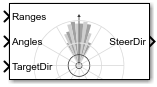Documentation

# Vector Field Histogram

Avoid obstacles using vector field histogram

• Library:
• Navigation Toolbox / Control Algorithms## Description

The Vector Field Histogram (VFH) block enables your vehicle to avoid obstacles based on range sensor data. Given a range sensor reading in terms of ranges and angles, and a target direction to drive toward, the VFH controller computes an obstacle-free steering direction.

For more information on the algorithm details, see Vector Field Histogram under Algorithms.

## Limitations

• The `Ranges` and `Angles` inputs are limited to 4000 elements when generating code for models that use this block.

## Ports

### Input

expand all

Range values from scan data, specified as a vector of scalars in meters. These range values are distances from a sensor at specified angles. The vector must be the same length as the corresponding Angles vector.

Angle values from scan data, specified as a vector of scalars in radians. These angle values are the specific angles of the specified ranges. The vector must be the same length as the corresponding Ranges vector.

Target direction for the vehicle, specified as a scalar in radians. The forward direction of the vehicle is considered zero radians, with positive angles measured counterclockwise. You can use the TargetDir output from the Pure Pursuit block when generating controls from a set of waypoints.

### Output

expand all

Steering direction for the vehicle, specified as a scalar in radians. This obstacle-free direction is calculated based on the VFH+ algorithm. The forward direction of the vehicle is considered zero radians, with positive angles measured counterclockwise.

## Parameters

expand all

#### Main

Number of bins used to create the histograms, specified as a scalar. This parameter is nontunable. You can set this parameter only when the object is initialized.

Limits for range readings in meters, specified as a two-element vector of scalars. The range readings input are only considered if they fall within the distance limits. Use the lower distance limit to ignore false positives from poor sensor performance at lower ranges. Use the upper limit to ignore obstacles that are too far away from the vehicle.

Thresholds for computing binary histogram, specified as a two-element vector of scalars. The algorithm uses these thresholds to compute the binary histogram from the polar obstacle density. Polar obstacle density values higher than the upper threshold are represented as occupied space (1) in the binary histogram. Values smaller than the lower threshold are represented as free space (0). Values that fall between the limits are set to the values of a previous computed binary histogram if one exists from previous iterations. If a previous histogram does not exist, the value is set as free space (0).

Radius of the vehicle, specified as a scalar in meters. This dimension defines the smallest circle that can circumscribe your vehicle. The vehicle radius is used to account for vehicle size when computing the obstacle-free direction.

Safety distance left around the vehicle position in addiction to vehicle radius, specified as a scalar in meters. The vehicle radius and safety distance are used to compute the obstacle-free direction.

Minimum turning radius for the vehicle moving at its current speed, specified as a scalar in meters.

• `Code generation` — Simulate model using generated C code. The first time you run a simulation, Simulink® generates C code for the block. The C code is reused for subsequent simulations, as long as the model does not change. This option requires additional startup time but the speed of the subsequent simulations is comparable to `Interpreted execution`.

• `Interpreted execution` — Simulate model using the MATLAB® interpreter. This option shortens startup time but has a slower simulation speed than ```Code generation```. In this mode, you can debug the source code of the block.

Tunable: No

#### Cost Function Weights

Cost function weight for moving toward the target direction, specified as a scalar. To follow a target direction, set this weight to be higher than the sum of Current direction weight and Previous direction weight. To ignore the target direction cost, set this weight to `0`.

Cost function weight for moving the vehicle in the current heading direction, specified as a scalar. Higher values of this weight produce efficient paths. To ignore the current direction cost, set this weight to `0`.

Cost function weight for moving in the previously selected steering direction, specified as a scalar. Higher values of this weight produce smoother paths. To ignore the previous direction cost, set this weight to `0`.

## Algorithms

expand all

### Topics

#### Introduced in R2019b